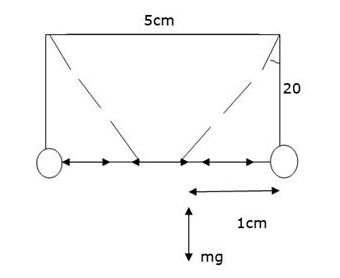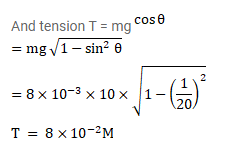# Two identical pith balls are charged by rubbing against each other.Question:

Two identical pith balls are charged by rubbing against each other. They are suspended from a horizontal rod through two strings of length $20 \mathrm{~cm}$ each, the separation between the suspension points being $5 \mathrm{~cm}$. In equilibrium, the separation points between the balls is $3 \mathrm{~cm}$. Find the mass of each ball has a magnitude $2.0 \times 10^{-8} \mathrm{c}$.

Solution: